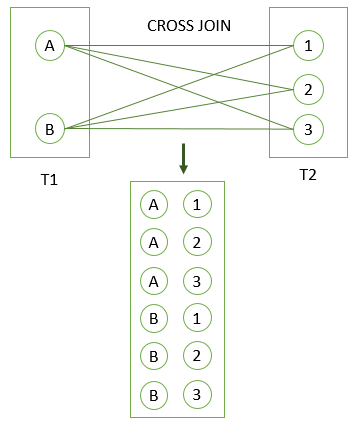# PostgreSQL Tutorial: Cross Join

August 3, 2023

Summary: in this tutorial, you will learn how to use the PostgreSQL `CROSS JOIN` to produce a cartesian product of rows from the joined tables.

## Introduction to the PostgreSQL CROSS JOIN clause

A `CROSS JOIN` clause allows you to produce a Cartesian Product of rows in two or more tables.

Different from other join clauses such as LEFT JOIN or INNER JOIN, the `CROSS JOIN` clause does not have a join predicate.

Suppose you have to perform a `CROSS JOIN` of two tables T1 and T2.

If T1 has `n` rows and T2 has `m` rows, the result set will have `nxm` rows. For example, the T1 has `1,000` rows and T2 has `1,000` rows, the result set will have `1,000 x 1,000` = `1,000,000` rows.

The following illustrates the syntax of the `CROSS JOIN` syntax:

``````SELECT select_list
FROM T1
CROSS JOIN T2;
``````

The following statement is equivalent to the above statement:

``````SELECT select_list
FROM T1, T2;
``````

Also, you can use an `INNER JOIN` clause with a condition that always evaluates to true to simulate the cross-join:

``````SELECT *
FROM T1
INNER JOIN T2 ON true;
``````

## PostgreSQL CROSS JOIN example

The following CREATE TABLE statements create T1 and T2 tables and insert some sample data for the cross-demonstration.

``````DROP TABLE IF EXISTS T1;
CREATE TABLE T1 (label CHAR(1) PRIMARY KEY);

DROP TABLE IF EXISTS T2;
CREATE TABLE T2 (score INT PRIMARY KEY);

INSERT INTO T1 (label)
VALUES
('A'),
('B');

INSERT INTO T2 (score)
VALUES
(1),
(2),
(3);
``````

The following statement uses the `CROSS JOIN` operator to join table T1 with table T2.

``````SELECT *
FROM T1
CROSS JOIN T2;
``````
`````` label | score
-------+-------
A     |     1
B     |     1
A     |     2
B     |     2
A     |     3
B     |     3
(6 rows)
``````

The following picture illustrates the result of the `CROSS JOIN` when joining the table T1 to the table T2:In this tutorial, you have learned how to use the PostgreSQL CROSS JOIN clause to make a Cartesian Product of rows in two or more tables.